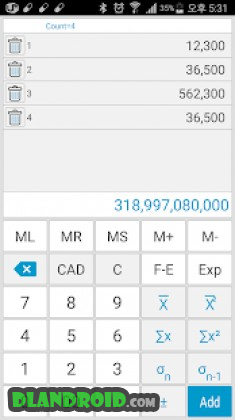# Total Calculator-Paid 9.4 Apk latest

event_note31 Oct 2018
android Apps, Tools

Total Calculator-Paid 9.4 Apk latest is a Tools Android app

Total Calculator-Paid is a Tools android app made by woosungsft that you can install on your android devices an enjoy !

★ Convenient and smart calculator with built-in function that can use various calculator in
one app and prevent user’s mistake as much as possible
★ History, memory storage function, Undo, Redo function
★ Supports 7 different calculator themes
★ Calculator button sound and font change function

– Integrated calculator configuration –

1) Simple Calculator
1) General Calculator
2) Engineering Calculator
3) Statistical calculator
4) Precision Calculator
5) Date Calculator
6) Unit conversion
7) Percent Calculator
8) Interest calculator (deposit / savings / loan)Total Calculator-Paid ApkTotal Calculator-Paid Apk

Whats New:

☞ ver 9.4
→ Memory function improvement
→ Undo, read function error correctio